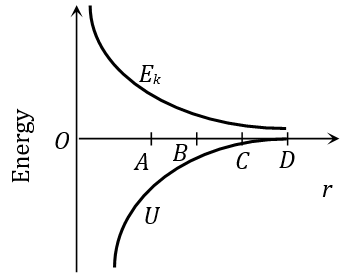Motion of planets and satellites
Question

# The curves for potential energy (U) and kinetic energy $\left({E}_{k}\right)$ of a two particle system are shown in figure. At what points the system will be bound?Moderate
Solution

## The system will be bound at points where total energy is negative. In the given curve at point A, B and C the P.E. is more than K.E.

Get Instant Solutions